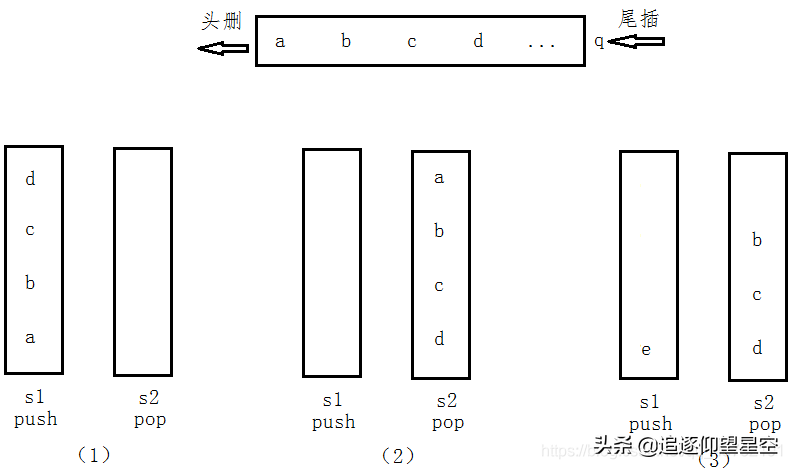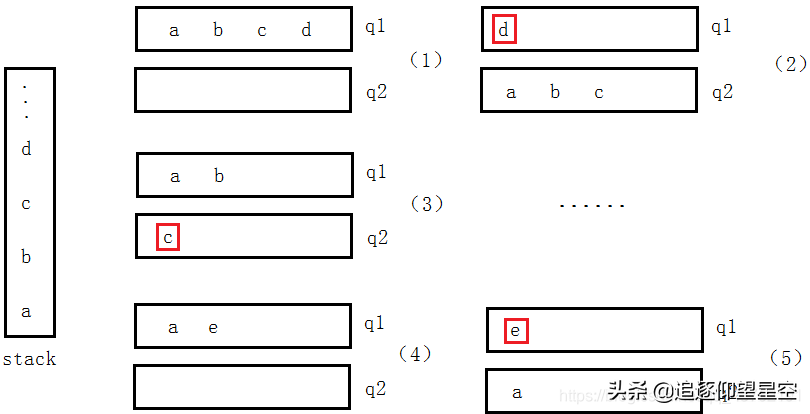# 脑筋急转弯：如何用两个栈实现一个队列？用两个队列实现一个栈 (1)• 也省得倒腾来倒腾去。

• 如果两个队列都被清空了，我们就再次将标兵 A 置为-1，这样还能提高代码的复用性。

02 别人的想法

========

==========template<typename T> class CQueue

{

public:

CQueue(void);

~CQueue(void);

void appendTail(const T& node);

private:

stack<T> stack1;

stack<T> stack2;

};

template<typename T>

void CQueue<T>::appendTail(const& T& element)//尾插

{

stack1.push(element);

}

template<typename T>

{

if (stack2.size() <= 0)

{

while (stack1.size > 0)

{

T& data = stack1.top();

stack1.pop();

stack2.push(data);

}

}

stack2.pop();

if (stack2.size() == 0)//当 stack2 为空时，抛异常

throw new exception("queue is empty");

}

============【一线大厂Java面试题解析+核心总结学习笔记+最新架构讲解视频+实战项目源码讲义】浏览器打开：qq.cn.hn/FTf 免费领取

bc”从 q1 中头删，然后再 q2 中尾插进来之后，头删 q1 中的元素“d”，就相当于实现了栈顶元素的出栈；

template<typename T> class CStack

{

public:

CStack(void);

~CStack(void);

void appendTail(const T& node);

private:

queue<T> q1;

queue<T> q2;

};

template<typename T>

void CStack<T>::appendTail(const T& node)//实现栈元素的插入

{

//数据的插入原则：保持一个队列为空，一个队列不为空，往不为空的队列中插入元素

if (!q1.empty())

{

q1.push(node);

}

else

{

q2.push(node);

}

}## 评论